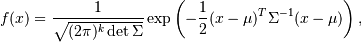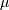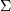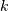# scipy.stats.multivariate_normal¶

scipy.stats.multivariate_normal = <scipy.stats._multivariate.multivariate_normal_gen object at 0x2b45d3298990>[source]

A multivariate normal random variable.

The mean keyword specifies the mean. The cov keyword specifies the covariance matrix.

New in version 0.14.0.

Parameters: x : array_like Quantiles, with the last axis of x denoting the components. mean : array_like, optional Mean of the distribution (default zero) cov : array_like, optional Covariance matrix of the distribution (default one) Alternatively, the object may be called (as a function) to fix the mean and covariance parameters, returning a “frozen” multivariate normal random variable: rv = multivariate_normal(mean=None, scale=1) Frozen object with the same methods but holding the given mean and covariance fixed.

Notes

Setting the parameter mean to None is equivalent to having mean be the zero-vector. The parameter cov can be a scalar, in which case the covariance matrix is the identity times that value, a vector of diagonal entries for the covariance matrix, or a two-dimensional array_like.

The covariance matrix cov must be a (symmetric) positive semi-definite matrix. The determinant and inverse of cov are computed as the pseudo-determinant and pseudo-inverse, respectively, so that cov does not need to have full rank.

The probability density function for multivariate_normal iswhereis the mean,the covariance matrix, andis the dimension of the space wheretakes values.

Examples

>>> from scipy.stats import multivariate_normal
>>> x = np.linspace(0, 5, 10, endpoint=False)
>>> y = multivariate_normal.pdf(x, mean=2.5, cov=0.5); y
array([ 0.00108914,  0.01033349,  0.05946514,  0.20755375,  0.43939129,
0.56418958,  0.43939129,  0.20755375,  0.05946514,  0.01033349])
>>> plt.plot(x, y)


The input quantiles can be any shape of array, as long as the last axis labels the components. This allows us for instance to display the frozen pdf for a non-isotropic random variable in 2D as follows:

>>> x, y = np.mgrid[-1:1:.01, -1:1:.01]
>>> pos = np.empty(x.shape + (2,))
>>> pos[:, :, 0] = x; pos[:, :, 1] = y
>>> rv = multivariate_normal([0.5, -0.2], [[2.0, 0.3], [0.3, 0.5]])
>>> plt.contourf(x, y, rv.pdf(pos))


Methods

 pdf(x, mean=None, cov=1) Probability density function. logpdf(x, mean=None, cov=1) Log of the probability density function. rvs(mean=None, cov=1) Draw random samples from a multivariate normal distribution. entropy() Compute the differential entropy of the multivariate normal.

#### Previous topic

scipy.stats.wrapcauchy

#### Next topic

scipy.stats.bernoulli